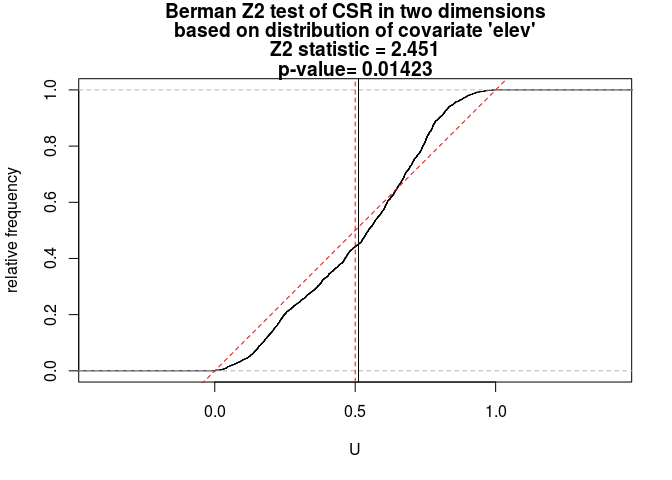# Lab 3: Intensity dependent on covariate

This session covers tools for investigating intensity depending on a covariate. The lecturer’s R script is available here (right click and save).

``````library(spatstat)
``````

### Exercise 1

The `bei` dataset gives the locations of trees in a survey area with additional covariate information in a list `bei.extra`.

1. Assign the elevation covariate to a variable `elev` by typing

``````elev <- bei.extra\$elev
``````

OK, lets do that:

``````elev <- bei.extra\$elev
``````
2. Plot the trees on top of an image of the elevation covariate.

``````plot(elev, main = "")
plot(bei, add = TRUE, cex = 0.3, pch = 16, cols = "white")
``````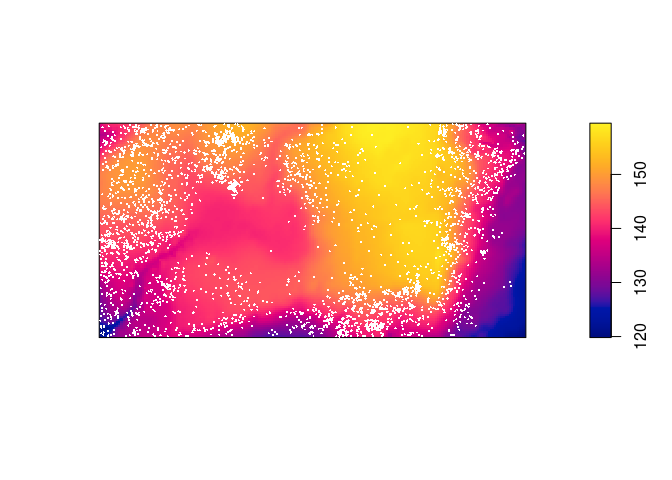3. Cut the study region into 4 areas according to the value of the terrain elevation, and make a texture plot of the result.

``````Z <- cut(elev, 4, labels=c("Low", "Med-Low", "Med-High", "High"))
textureplot(Z, main = "")
``````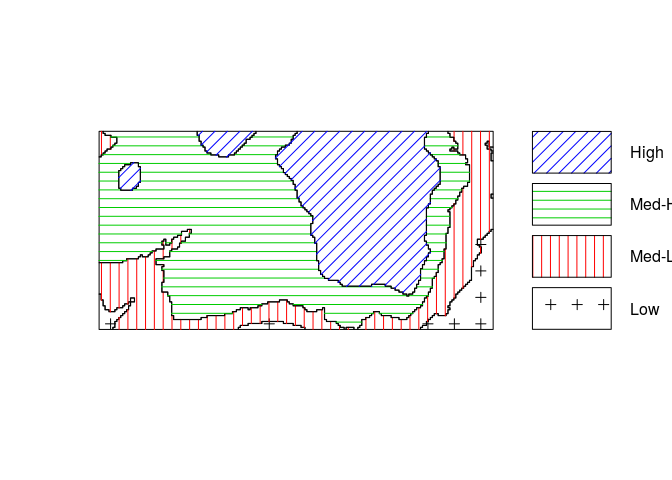4. Convert the image from above to a tesselation, count the number of points in each region using `quadratcount`, and plot the quadrat counts.

``````Y <- tess(image = Z)
qc <- quadratcount(bei, tess = Y)
``````
5. Estimate the intensity in each of the four regions.

``````intensity(qc)
``````
``````## tile
##         Low     Med-Low    Med-High        High
## 0.002259007 0.006372523 0.008562862 0.005843516
``````

### Exercise 2

Assume that the intensity of trees is a function λ(u)=ρ(e(u)) where e(u) is the terrain elevation at location u.

1. Compute a nonparametric estimate of the function ρ and plot it by

``````rh <- rhohat(bei, elev)
plot(rh)
``````

Repeating the R code:

``````rh <- rhohat(bei, elev)
plot(rh)
``````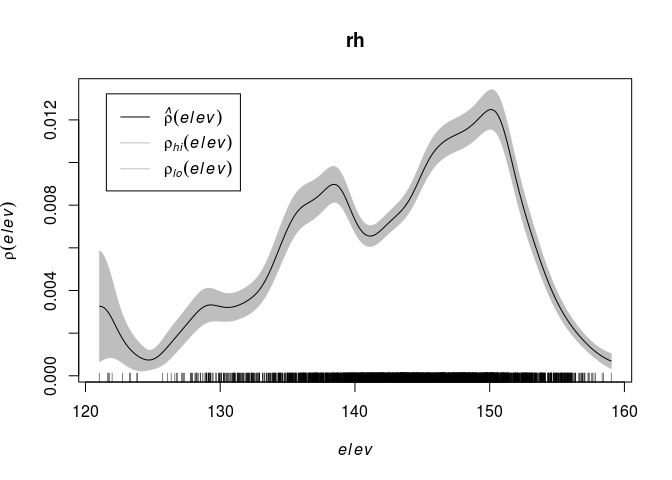2. Compute the predicted intensity based on this estimate of ρ.

``````prh <- predict(rh)
plot(prh, main = "")
plot(bei, add = TRUE, cols = "white", cex = .2, pch = 16)
``````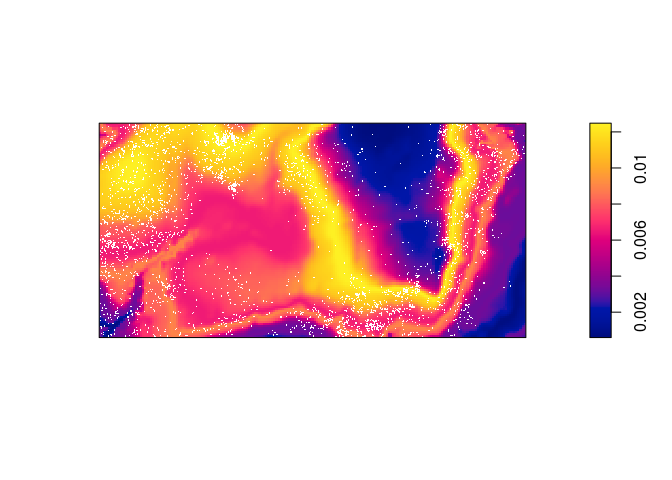3. Compute a non-parametric estimate by kernel smoothing and compare with the predicted intensity above.

The kernel density estimate of the points is computed and plotted with the following code:

``````dbei <- density(bei, sigma = bw.scott)
plot(dbei, main = "")
plot(bei, add = TRUE, cols = "white", cex = .2, pch = 16)
``````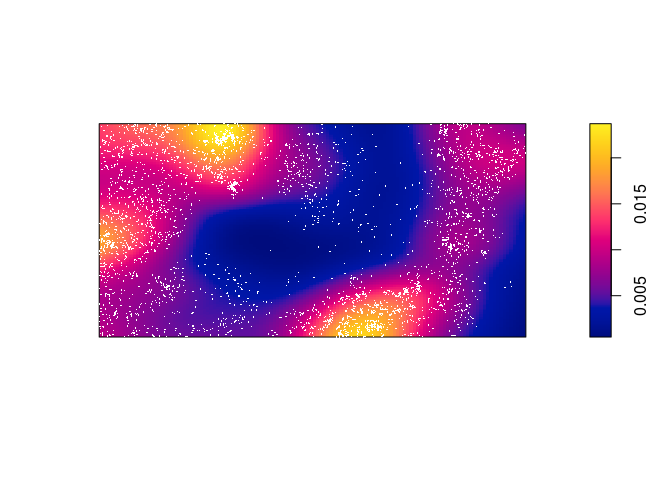Which seems to be quite different form the predicted intentisty.

4. Bonus info: To plot the two intensity estimates next to each other you collect the estimates as a spatial object list (`solist`) and plot the result (the estimates are called `pred` and `ker` below):

``````l <- solist(pred, ker)
plot(l, equal.ribbon = TRUE, main = "",
main.panel = c("rhohat prediction", "kernel smoothing"))
``````
``````l <- solist(prh, dbei)
plot(l, equal.ribbon = TRUE, main = "",
main.panel = c("rhohat prediction", "kernel smoothing"))
``````### Exercise 3

1. Continuing with the dataset `bei` conduct both Berman’s Z1 and Z2 tests for dependence on `elev`, and plot the results.

The tests are done straightforwardly with `berman.test`:

``````Z1 <- berman.test(bei, elev)
print(Z1)
``````
``````##
##  Berman Z1 test of CSR in two dimensions
##
## data:  covariate 'elev' evaluated at points of 'bei'
## Z1 = -0.72924, p-value = 0.4659
## alternative hypothesis: two-sided
``````
``````plot(Z1)
``````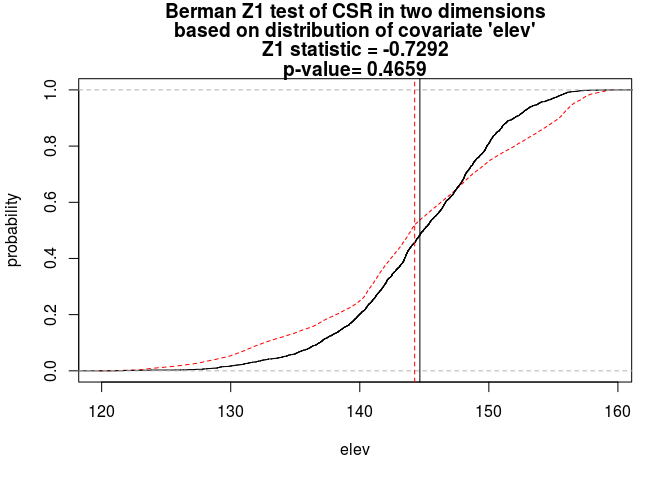``````Z2 <- berman.test(bei, elev, which = "Z2")
print(Z2)
``````
``````##
##  Berman Z2 test of CSR in two dimensions
##
## data:  covariate 'elev' evaluated at points of 'bei'
##   and transformed to uniform distribution under CSR
## Z2 = 2.4515, p-value = 0.01423
## alternative hypothesis: two-sided
``````
``````plot(Z2)
``````